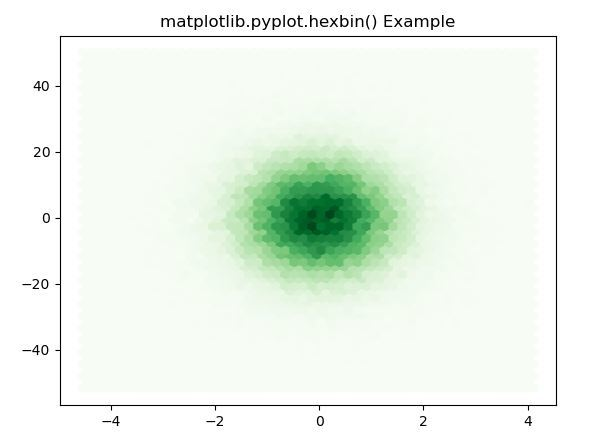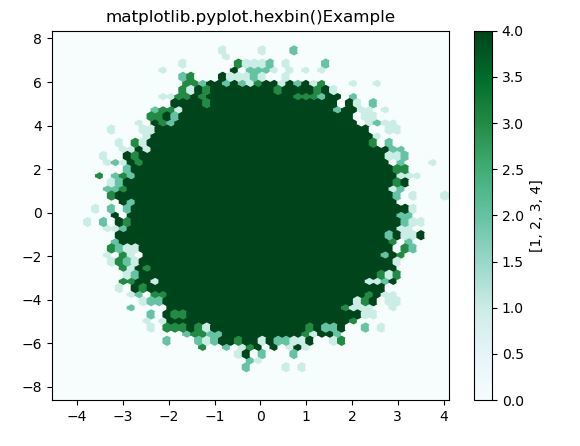Related Articles

# Matplotlib.pyplot.hexbin() function in Python

• Last Updated : 05 Jun, 2020

Matplotlib is a library in Python and it is numerical – mathematical extension for NumPy library. Pyplot is a state-based interface to a Matplotlib module which provides a MATLAB-like interface. There are various plots which can be used in Pyplot are Line Plot, Contour, Histogram, Scatter, 3D Plot, etc.

## Matplotlib.pyplot.hexbin() function

The hexbin() function in pyplot module of matplotlib library is used to make a 2D hexagonal binning plot of points x, y.

Syntax: matplotlib.pyplot.hexbin(x, y, C=None, gridsize=100, bins=None, xscale=’linear’, yscale=’linear’, extent=None, cmap=None, norm=None, vmin=None, vmax=None, alpha=None, linewidths=None, edgecolors=’face’, reduce_C_function=, mincnt=None, marginals=False, *, data=None, **kwargs)

Parameters: This method accept the following parameters that are described below:

• x, y: These parameter are the sequence of data. x and y must be of the same length.
• C : This parameter are the values which are accumulated in the bins.
• gridsize : This parameter represents the number of hexagons in the x-direction or both direction.
• xscale : This parameter uses a linear or log10 scale on the horizontal axis.
• xycale : This parameter uses a linear or log10 scale on the vertical axis.
• mincnt : This parameter is used to display cells with more than mincnt number of points in the cell.
• marginals : This parameter is used to plot the marginal density as colormapped rectangles along the bottom of the x-axis and left of the y-axis.
• extent : This parameter is the limits of the bins.

Returns: This returns the following:

• polycollection : This returns the PolyCollection defining the hexagonal bins.

Below examples illustrate the matplotlib.pyplot.hexbin() function in matplotlib.pyplot:

Example 1:

## Python3

 `# Implementation of matplotlib function ``import` `matplotlib.pyplot as plt ``import` `numpy as np ``   ` `np.random.seed(``19680801``) ``   ` `n ``=` `100000``x ``=` `np.random.standard_normal(n) ``y ``=` `12` `*` `np.random.standard_normal(n) ``    ` `plt.hexbin(x, y, gridsize ``=` `50``, cmap ``=``'Greens'``) ``plt.title(``'matplotlib.pyplot.hexbin() Example'``) ``plt.show() `

Output:Example 2:

## Python3

 `# Implementation of matplotlib function ``import` `matplotlib.pyplot as plt ``import` `numpy as np ``   ` `np.random.seed(``19680801``) ``   ` `n ``=` `100000``x ``=` `np.random.standard_normal(n) ``y ``=` `2` `*` `np.random.standard_normal(n) ``z ``=``[``1``, ``2``, ``3``, ``4``] ``xmin ``=` `x.``min``() ``xmax ``=` `x.``max``() ``ymin ``=` `y.``min``() ``ymax ``=` `y.``max``() ``   ` `hb ``=` `plt.hexbin(x, y, gridsize ``=` `50``, ``               ``bins ``=` `z, cmap ``=``'BuGn'``) ``   ` `plt.xlim(xmin, xmax)``plt.ylim(ymin, ymax)``   ` `cb ``=` `plt.colorbar(hb) ``cb.set_label(z)``plt.title('matplotlib.pyplot.hexbin()\``Example')`` ` `plt.show()`

Output:Attention geek! Strengthen your foundations with the Python Programming Foundation Course and learn the basics.

To begin with, your interview preparations Enhance your Data Structures concepts with the Python DS Course. And to begin with your Machine Learning Journey, join the Machine Learning – Basic Level Course

My Personal Notes arrow_drop_up